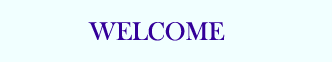TUTORIALS

## PHP Basic

PHP HOME
PHP Intro
PHP Install
PHP Syntax
PHP Variables
PHP String
PHP Operators
PHP If...Else
PHP Switch
PHP Arrays
PHP While Loops
PHP For Loops
PHP Functions
PHP Forms
PHP \$_GET
PHP \$_POST

PHP Date
PHP Include
PHP File
PHP Sessions
PHP E-mail
PHP Secure E-mail
PHP Error
PHP Exception
PHP Filter

## PHP Database

MySQL Introduction
MySQL Connect
MySQL Create
MySQL Insert
MySQL Select
MySQL Where
MySQL Order By
MySQL Update
MySQL Delete
PHP ODBC

XML Expat Parser
XML DOM
XML SimpleXML

AJAX Intro
AJAX PHP
AJAX Database
AJAX XML
AJAX Live Search
AJAX Poll

PHP Array
PHP Calendar
PHP Date
PHP Directory
PHP Error
PHP Filesystem
PHP Filter
PHP FTP
PHP HTTP
PHP Libxml
PHP Mail
PHP Math
PHP Misc
PHP MySQL
PHP SimpleXML
PHP String
PHP XML
PHP Zip

PHP Quiz
PHP Exam

# PHP Math Functions

## PHP Math Introduction

The math functions can handle values within the range of integer and float types.

## Installation

The math functions are part of the PHP core. There is no installation needed to use these functions.

## PHP Math Functions

PHP: indicates the earliest version of PHP that supports the function.

Function Description PHP
abs() Returns the absolute value of a number 3
acos() Returns the arccosine of a number 3
acosh() Returns the inverse hyperbolic cosine of a number 4
asin() Returns the arcsine of a number 3
asinh() Returns the inverse hyperbolic sine of a number 4
atan() Returns the arctangent of a number as a numeric value between -PI/2 and PI/2 radians 3
atan2() Returns the angle theta of an (x,y) point as a numeric value between -PI and PI radians 3
atanh() Returns the inverse hyperbolic tangent of a number 4
base_convert() Converts a number from one base to another 3
bindec() Converts a binary number to a decimal number 3
ceil() Returns the value of a number rounded upwards to the nearest integer 3
cos() Returns the cosine of a number 3
cosh() Returns the hyperbolic cosine of a number 4
decbin() Converts a decimal number to a binary number 3
dechex() Converts a decimal number to a hexadecimal number 3
decoct() Converts a decimal number to an octal number 3
exp() Returns the value of Ex 3
expm1() Returns the value of Ex - 1 4
floor() Returns the value of a number rounded downwards to the nearest integer 3
fmod() Returns the remainder (modulo) of the division of the arguments 4

getrandmax()

Returns the maximum random number that can be returned by a call to the rand() function 3
hexdec() Converts a hexadecimal number to a decimal number 3
hypot() Returns the length of the hypotenuse of a right-angle triangle 4
is_finite() Returns true if a value is a finite number 4
is_infinite() Returns true if a value is an infinite number 4
is_nan() Returns true if a value is not a number 4
lcg_value() Returns a pseudo random number in the range of (0,1) 4
log() Returns the natural logarithm (base E) of a number 3
log10() Returns the base-10 logarithm of a number 3
log1p() Returns log(1+number) 4
max() Returns the number with the highest value of two specified numbers 3
min() Returns the number with the lowest value of two specified numbers 3
mt_getrandmax() Returns the largest possible value that can be returned by mt_rand() 3
mt_rand() Returns a random integer using Mersenne Twister algorithm 3
mt_srand() Seeds the Mersenne Twister random number generator 3
octdec() Converts an octal number to a decimal number 3
pi() Returns the value of PI 3
pow() Returns the value of x to the power of y 3
rand() Returns a random integer 3
round() Rounds a number to the nearest integer 3
sin() Returns the sine of a number 3
sinh() Returns the hyperbolic sine of a number 4
sqrt() Returns the square root of a number 3
srand() Seeds the random number generator 3
tan() Returns the tangent of an angle 3
tanh() Returns the hyperbolic tangent of an angle 4

## PHP Math Constants

PHP: indicates the earliest version of PHP that supports the constant.

Constant Description PHP
M_E Returns e (approx. 2.718) 4
M_EULER Returns Euler's constant (approx. 0.577) 4
M_LNPI Returns the natural logarithm of PI (approx. 1.144) 4
M_LN2 Returns the natural logarithm of 2 (approx. 0.693) 4
M_LN10 Returns the natural logarithm of 10 (approx. 2.302) 4
M_LOG2E Returns the base-2 logarithm of E (approx. 1.442) 4
M_LOG10E Returns the base-10 logarithm of E (approx. 0.434) 4
M_PI Returns PI (approx. 3.14159) 3
M_PI_2 Returns PI/2 (approx. 1.570) 4
M_PI_4 Returns PI/4 (approx. 0.785) 4
M_1_PI Returns 1/PI (approx. 0.318) 4
M_2_PI Returns 2/PI (approx. 0.636) 4
M_SQRTPI Returns the square root of PI (approx. 1.772) 4
M_2_SQRTPI Returns 2/square root of PI (approx. 1.128) 4
M_SQRT1_2 Returns the square root of 1/2 (approx. 0.707) 4
M_SQRT2 Returns the square root of 2 (approx. 1.414) 4
M_SQRT3 Returns the square root of 3 (approx. 1.732) 4